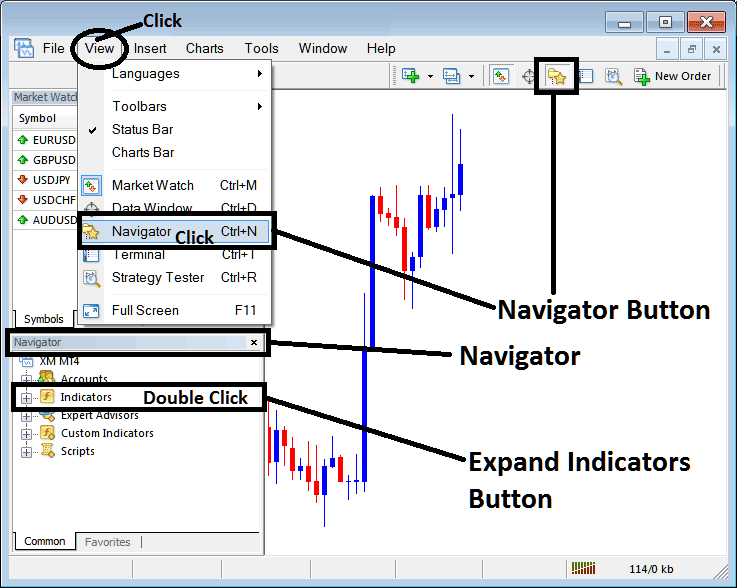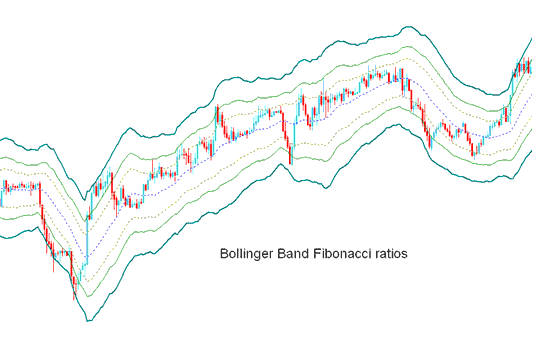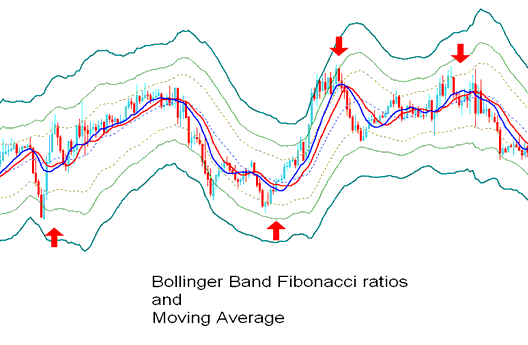## Step 1: Open the Indices Trading Platform Navigator Window

Open the Navigator window as shown below; Go to “View” menu (click), then select the “Navigator” window (click), or From Standard Toolbar click the “Navigator” button or press short cut keyboard keys “Ctrl+N”

On the Navigator window, select “Indicators”, (Double Click)MetaTrader 4 Bollinger Bands - Fibonacci Ratios Indices Indicator

Expand the menu by clicking the expand button marker (+) or double click the “indicators” menu, after this the button will appear as (-) and will now display a list as shown below - select the Bollinger Bands - Fibonacci Ratios stock indices chart indicator from this list of stock indices indicators so as to add the Bollinger Bands - Fibonacci Ratios stock indexes technical indicator on the stock indexes trading chart.From the Above window you can then place Bollinger Bands - Fibonacci Ratios indicator that you want on the Indices Trading chart

## How to Put Custom Bollinger Bands

If the stock indices indicator you want to add is a custom indicator - for example if the Bollinger Bands - Fibonacci Ratios indicator you want to add is a custom indicator you will need to first add this custom Bollinger Bands - Fibonacci Ratios indicator on the MT4 stock indexes trading platform and the compile the custom Bollinger Bands - Fibonacci Ratios indicator so that the newly added Bollinger Bands - Fibonacci Ratios custom indicator appear on the list of custom indicators on the MetaTrader 4 stock indexes trading platform.

To learn how to install Bollinger Bands - Fibonacci Ratios indicators on MetaTrader 4, how to add Bollinger Bands - Fibonacci Ratios indicator window to MetaTrader 4 and how to add Bollinger Bands - Fibonacci Ratios custom indicator on MT4 stock indexes trading platform - How to add a custom Bollinger Bands - Fibonacci Ratios indices trading Indicator on MT4.

# Bollinger Bands

Derived from the original Bollinger bands.

The Bollinger Fibonacci ratios is a volatility based indicators but it does not use the standard deviation to calculate the width of the bands instead it uses a smoothed ATR that are multiplied with Fibonacci ratios of 1.618, 2.618, and 4.236.

The smoothed lines that are multiplied with Fibonacci ratios are then added or subtracted from the moving average.

This forms 3 upper Fibonacci bands and 3 lower Fibonacci bands

The middle band forms the basis of the trend.## Indices Technical Analysis and Generating Indices Trading Signals

This indicator used to determine point of support and resistance for indices.

The lines below represent support points while those above are resistance levels.

The outermost bands provide the strongest resistance/support.

The inner most bands provide least support/resistance.

The innermost band represents Fibonacci 38.2 % retracement level

The second band represents Fibonacci 50 % retracement level

The outermost band represents Fibonacci 61.8 % retracement level

The indicator is used to determine points where stock indexes price might reverse. (Indices Price Pullback Levels)

When stock indexes price hits one of the lines and reverses then an entry or exit signal is generated.

However, it is always good to combine the signal with other confirmation indicators such as the moving average to confirm the signal as shown below.Technical Analysis